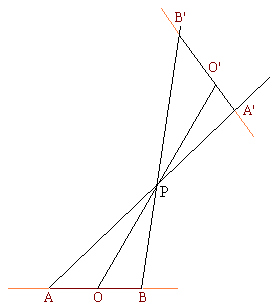## Geometric construction with the straightedge alone

It's impossible to find the center of a given circle with the straightedge alone.

This is a Jacob Steiner's theorem. The proof will follow that of David Hilbert.

Let's fix a circle C. The one I selected lies in the plane perpendicular to the page and has one diameter denoted AB. The plan is to find a transformation T of the plane such that, under T, straight lines remain straight lines and our circle (the curve) stays unchanged. However, the transformation we are looking for will not preserve the center of the circle.For now assume such a tranformation T indeed exists. Assume also that there exists a construction that uses only a straightedge that, according to the construction rules, produces a sequence of straight lines such that at the end two of the lines intersect at the center of the circle. As we draw lines from the sequence we may as well draw their images under T. These image lines will follow the construction rules as well, but, as the result, will produce a different point. Which is of course absurd.

Now let's turn to the transformation T. On the diagram, it's a projective transformation between two copies of the same plane. The circle C is carried into the circle with diameter A'B'. Obviously, the centers O and O' do not correspond to one another.

## References

1. R. Courant and H. Robbins, What is Mathematics?, Oxford University Press, 1996
2. M. Kac and S. M. Ulam, Mathematics and Logic, Dover Publications, NY, 1968.
3. H. Rademacher and O. Toeplitz, The Enjoyment of Mathematics, Dover Publications, 1990.•